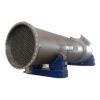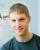4 votes

# Shell And Tube Heat Exchanger Cost EstimationAs promised in my earlier blog entry "Cost Estimation Of Fixed Roof (Cone) Carbon Steel Storage Tanks", today's blog entry relates to cost estimation of S&T heat exchangers. The equations are empirical and the reference is the same i.e.:

"Estimate Costs of Heat Exchangers and Storage Tanks via Correlations" by Armando B. Corripio, Katherine S. Chrien, and Lawrence B. Evans, Chemical Engineering magazine January 25, 1982.

There is a bunch of equations and it took some time to program it in excel but ultimately it was done.

Let us get down to the equations:

Base Cost for CS Floating-Head, 700 kPag (100 psig) exchanger

SI Units

CB = EXP(8.202 + 0.01506*lnA + 0.06811*(lnA)2)

where:

CB = Base Cost of the HE, USD

A = Heat Transfer Area in m2

Lower Limit: 14 m2; Upper Limit: 1100 m2

Exchanger-type cost factor, FD

FD = EXP(-0.9003 + 0.0906*lnA)

Kettle Reboiler:

FD = 1.35

U-Tube:

FD = EXP(-0.7844 + 0.0830*lnA)

where:

FD = Exchanger-type cost factor

Design-pressure cost factor, FP

700-2100 kPag:

FP = 0.8955 + 0.04981*lnA

2100-4200 kPag:

FP = 1.2002 + 0.07140*lnA

4200-6200 kPag:

FP = 1.4272 + 0.12088*lnA

where:

FP = Design-pressure cost factor

Material-of-Construction Cost Factor, FM

SS316:

FM =1.4144 + 0.23296*lnA

SS304:

FM =1.1991 + 0.15984*lnA

SS347:

FM =1.1388 + 0.22186*lnA

Nickel 200:

FM =2.9553 + 0.60859*lnA

Monel 400:

FM =2.3296 + 0.43377*lnA

Inconel 600:

FM =2.4103 + 0.50764*lnA

Incoloy 825:

FM =2.3665 + 0.49706*lnA

Titanium:

FM =2.5617 + 0.42913*lnA

Hastelloy:

FM =3.7614 + 1.51774*lnA

where:

FM = Material-of-construction cost factor

Heat-Exchanger Cost , CE

CE = CB*FB*FD*FM

where:

CE = Heat Exchanger Cost, USD

USC Units

CB = EXP(8.551 - 0.30863*lnA + 0.06811*(lnA)2)

where:

CB = Base Cost of the HE, USD

A = Heat Transfer Area in ft2

Lower Limit: 150 ft2; Upper Limit: 12000 ft2

Exchanger-type cost factor, FD

FD = EXP(-1.1156 + 0.0906*lnA)

Kettle Reboiler:

FD = 1.35

U-Tube:

FD = EXP(-0.9816 + 0.0830*lnA)

where:

FD = Exchanger-type cost factor

Design-pressure cost factor, FP

100-300 psig:

FP = 0.7771 + 0.04981*lnA

300-600 psig:

FP = 1.0305 + 0.07140*lnA

600-900 psig:

FP = 1.14 + 0.12088*lnA

where:

FP = Design-pressure cost factor

Material-of-Construction Cost Factor, FM

SS316:

FM =0.8608 + 0.23296*lnA

SS304:

FM =0.8193 + 0.15984*lnA

SS347:

FM =0.6116 + 0.22186*lnA

Nickel 200:

FM =1.5092 + 0.60859*lnA

Monel 400:

FM =1.2989 + 0.43377*lnA

Inconel 600:

FM =1.2040 + 0.50764*lnA

Incoloy 825:

FM =1.1854 + 0.49706*lnA

Titanium:

FM =1.5420 + 0.42913*lnA

Hastelloy:

FM =0.1549 + 1.51774*lnA

where:

FM = Material-of-construction cost factor

Heat-Exchanger Cost , CE

CE = CB*FB*FD*FM

where:

CE = Heat Exchanger Cost, USD

Updated Heat Exchanger Cost

CE(updated) = (CI(calculation yr) / CI(base yr)) * CE

where:

CI(calculation yr) = cost Index for the calculation year &

CI(base yr) = cost index for the base year

Notes:

1. As an example, if you are trying to calculate the cost of the exchanger for the year 2010, the most probable updated cost would be based on a ratio of the cost index for the year 2010 to the cost index for a base year of 2005. It is not recommended to use a base cost index more than 5 years older than the cost index for the calculated year.

2. The cost index ratio is subjective in nature and readers are exercised caution in determining the "Cost Index Ratio" for calculating the updated equipment cost.

Readers and Members of "Cheresources", this is it for today's blog entry. Would welcome comments from all of you on the subject matter.

Regards,
Ankur.Prafulla
Thanks Very Useful Sirdodozn
Hi I would like to know which year these equations apply to so that I can use the appropriate cost index.ankur2061

Hi I would like to know which year these equations apply to so that I can use the appropriate cost index.

These equations are based on an article published in "Chemical Engineering" in 1982. However, you cannot simply use a number for cost index on a pro-rata basis from the year 1982 up to the current year. This will be the tricky part i.e. to arrive at the ratio of the cost-index for the year of consideration to the base year.joker1
Ankur,
The Heat exchanger cost equation refers to Fb which I cannot find. Are you referring to Fp (design pressure cost factor) ?ankur2061

Ankur,
The Heat exchanger cost equation refers to Fb which I cannot find. Are you referring to Fp (design pressure cost factor) ?

Yes, FB is a typo error and should be read as FP. Thanks for the correction.

Regards,
Ankurciwstevie
Nice post, always good to have some tools for cost calculations at hands...but....

Isn't it a bit strange to give a cost correlation dating from 1982 and then stating that the CEPCI should be used to update costs from max. 5 years back only? Would be interesting to compare the results to other (freely available) cost estimation sites. See links below:

Peters/Timmerhaus: http://www.mhhe.com/...al/peters/data/
Matches: http://www.matche.co...pCost/index.htmT.S.MURALI
Trust this compares well with a manual based excel program which will take into
account all the factors . And also the formulae of other publications
-Walace ,Timmerhaus etc.
Thanks for the useful and handy tool presented.Amit1563

Sir,

Thanks a lot for these correlations. Would like to know your opinion on how the shell inner diameter would affect the cost of heat exchanger. I feel it could play a significant role. Moreover if you could provide a link to the softcopy of the reference, it would be highly appreciated.ojimenezp

Very useful info!George-Florin

Hello Mr.Ankur, I canot find the excel.is it still posted here?

Here is my e-mail address if you would like to send me

prunaru.florin@gmail.com

Thanks!Raj Mehta

Can I use the empirical correlation if my area is below the lower limit ? If not, then how can I calculate the cost?

### Latest Visitors

•englobaljon
Today, 11:33 AM
•dhavali
Today, 02:24 AM
•alexpage88
Yesterday, 04:24 AM
•sas969
Yesterday, 01:25 AM
•nddallal
05 Oct 2022 - 19:04
•sehgalvipin
05 Oct 2022 - 12:59
•prasanthp1313
05 Oct 2022 - 11:04
•rcalaforrall
05 Oct 2022 - 10:06
•Promer2
05 Oct 2022 - 09:44